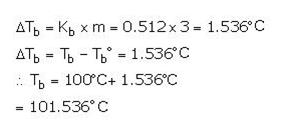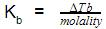Courses

# Test: Boiling And Freezing Points Of Solutions

## 15 Questions MCQ Test Chemistry for JEE | Test: Boiling And Freezing Points Of Solutions

Description
This mock test of Test: Boiling And Freezing Points Of Solutions for JEE helps you for every JEE entrance exam. This contains 15 Multiple Choice Questions for JEE Test: Boiling And Freezing Points Of Solutions (mcq) to study with solutions a complete question bank. The solved questions answers in this Test: Boiling And Freezing Points Of Solutions quiz give you a good mix of easy questions and tough questions. JEE students definitely take this Test: Boiling And Freezing Points Of Solutions exercise for a better result in the exam. You can find other Test: Boiling And Freezing Points Of Solutions extra questions, long questions & short questions for JEE on EduRev as well by searching above.
QUESTION: 1

### Which one of the following is not a colligative property?

Solution:

Depression in freezing point is a colligative property but freezing point is not a colligative property.

QUESTION: 2

### The molal elevation constant is the elevation in boiling point of

Solution:

The correct answer is Option B.

Molal elevation constant is a characteristic constant for a given solvent. It is the elevation of boiling point produced when one mole of solute is dissolved in 1 kg of solvent. The proportionality constant, Kb, is called the molal boiling point elevation constant. It is a constant that is equal to the change in the boiling point for a 1-molal solution of a nonvolatile molecular solute.

QUESTION: 3

### The colligative properties of a solution are

Solution:

The correct answer is option A
The colligative properties of a solution are
Proportional to the number of solute particles present.
In chemistry, colligative properties are those properties of solutions that depend on the ratio of the number of solute particles to the number of solvent molecules in a solution, and not on the nature of the chemical species present.

QUESTION: 4

The information that is/are needed to determine the molar mass of an unknown solute is/are

Solution:
QUESTION: 5

What is the boiling point of a 3 molal aqueous solution if Kb is 0.512°C/m?

Solution:QUESTION: 6

Which of the following is a colligative property?

Solution:

Colligative properties- The properties that depend upon the ratio of the number of solute molecules and total molecules not upon the nature of solute molecules named as colligative properties.

Example- Osmotic pressure, elevation of boiling point, depression in freezing point and relative lowering of vapour pressure.

QUESTION: 7

Beckmann thermometers are used to measure

Solution:

The correct answer is Option B.
A Beckmann's thermometer is a device used to measure elevation in boiling point or depression in freezing point.

QUESTION: 8

A solution was made by dissolving 2 g of a solute in 100 g of acetone. The solution boiled at 56.95° C. The boiling point of pure acetone is 55.95° C, and the Kb =1.71°C/m. What is the molecular weight of the solute?

Solution:

The correct answer is Option B.

∆Tb =(Kb × 1000 × W2) / M2 × W1
⇒ M2 = (Kb × 1000 × W2) / ∆Tb × W1
= (1.71 × 1000 × 2) / 1 × 100
= 34.2g

QUESTION: 9

The molal elevation constant is the ratio of the elevation in B.P to:

Solution:

The correct answer is option A
Due to solute presence in the solution, the solution's boiling point is greater than the pure solvent's boiling point. This is called as elevation in boiling point and it can be represented as:
ΔTb​=Kb​ × molalityQUESTION: 10

Which of the two has lower freezing point, 2m NaCl or 5m NaCl aqueous solution?

Solution:

The correct answer is option C
ΔTf= Kf  x m Since ΔTf for 5m NaCl will be higher than for 2m, 5m NaCl solution freezes at a lower temperature.

QUESTION: 11

If the vapour pressure of pure solvent A is 17.5 mm and lowering of vapour pressure of solution formed by adding a non-volatile electrolyte is 0.0175 mm then what is the relative lowering of vapour pressure?

Solution:

The correct answer is option C
Relative lowering of vapour pressure:
= lowering of vapour pressure of solution/ vapour pressure of pure solvent
=0.0175/17.5=0.001

QUESTION: 12

Which of the following unit of concentration is independent of temperature?

Solution:

Molality (amount of moles per 1 kg of the solvent, mol/kg) and mole fraction (mol/mol) will remain constant since they are independent of volume of the solution which changes with temperature. Molarity i.e. amount of moles of a substance in 1 liter of a solution will change with volume of the solution so it will change with temperature.

QUESTION: 13

Relative lowering of vapour pressure is directly proportional to

Solution:

Relative lowering of vapour pressure is directly proportional to mole fraction of solute. Hence it is a colligative property.

QUESTION: 14

When common salt is dissolved in water:

Solution:

The correct answer is Option B.

When a non-volatile solute is dissolved in a pure solvent, its vapour pressure is decreased. The difference in the boiling points of the solution and pure solvent is called elevation in boiling point.

The elevation in the boiling point of a solution of nonelectrolyte is proportional to its molality and the equimolal solution of all the substances in the same solvent will show an equal elevation in boiling points. These are known as Raoult's laws of elevation of the boiling point.

So, the addition of salt will increase the boiling point of the solution.

QUESTION: 15

Camphor is used as solvent to determine the molecular mass of non-volatile solute by Rast method because for camphor

Solution:

The correct answer is “molal depression constant is high”.

Camphor is used for the determination of molecular masses of solute by Rast method because its molal depression constant is very high 40Kmolality-1 .